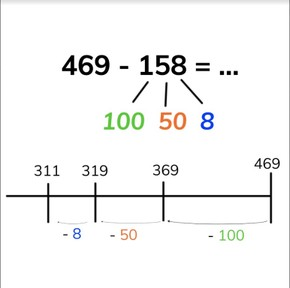Subtraction to 1,000 splitting the subtrahend

# Subtraction to 1,000 splitting the subtrahend

No account needed.8,000 schools use Gynzy92,000 teachers use Gynzy1,600,000 students use Gynzy

## General

Students learn to subtract numbers to 1,000 by splitting the subtrahend (the second number) into hundreds, tens and ones and taking those away from the first number (minuend) in the subtraction problem.

2.NBT.B.7

## Relevance

Discuss with students that it is useful to know how to split the second number (subtrahend) into different parts to make subtraction easier.

## Introduction

Show the maze on the interactive whiteboard. At every turn in the maze, students must solve the given math problem. If they solve each problem correctly they should reach the exit of the maze! Next give students a story problem and ask them to determine the answer individually or in pairs.

## Development

First explain to students that when splitting the subtrahend, you always split the subtrahend (the second number) into hundreds, tens, and ones and take them away separately from the first number (the minuend). Remind students that hundreds are a group of one hundred and end with zeros in the tens and ones places, that tens are a group of ten with a zero in the ones place, and that ones are the numbers from 1 to 9. Next explain to students that you can also subtract by splitting the subtrahend using the number line. The first number of the subtraction problem (minuend) goes at the end of the number line. Discuss that you can take jumps to take away the second number (the subtrahend). Show this to the students splitting the subtrahend into hundreds, tens and ones and jumping that on the number line. Ask students which number you jumped to on the number line. That number is your difference. Then explain to students that you can also subtract splitting the subtrahend without using a number line by splitting the subtrahend into hundreds, tens, and ones, and then taking them away from the first number in steps. You first take away the hundreds. You then take the tens away from the difference you found. Then you take the ones away from that difference to find the final difference. Ask students which steps they take in subtracting the given problems on the interactive whiteboard. Ask students to explain their strategy. Erase the grey boxes to check the answers.

Check that students are able to subtract by splitting the subtrahend by asking the following questions:
- What does it mean to split the subtrahend?
- What steps do you take in subtracting by splitting?
- How do you solve the following problems? 470 - 60 = ? and 364 - 41 = ?

## Guided practice

Students are given subtraction problems in which they are asked to jump back to a given number to discover how many are taken away. They are also given subtraction problems in which the subtrahend is already split, and problems in which they must determine how to split the subtrahend.

## Closing

Discuss with students that it is important to be able to subtract numbers to 1,000 splitting the subtrahend to make it easier to subtract. On the interactive whiteboard, show the child making a statement. Students must determine if their statement is correct or incorrect. If the students say that the statement is incorrect, ask them what the correct statement should be. You may choose to have students solve each problem presented by the children on the interactive whiteboard.

## Teaching tips

Students who have difficulty with this learning goal can make use of a number line. Have them split the subtrahend into hundreds, tens and ones, and then draw those jumps on the number line from the first number which they have at the end of the number line.

### The online teaching platform for interactive whiteboards and displays in schools

• Save time building lessons

• Manage the classroom more efficiently

• Increase student engagement